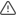## Introduction

The saturating math hyperfunctions help you perform saturating math on integers. In saturating math, the final result is bounded. If the result of a normal mathematical operation exceeds either the minimum or maximum bound, the result of the corresponding saturating math operation is capped at the bound. For example, `2 + (-3) = -1`. But in a saturating math function with a lower bound of `0`, such as `saturating_add_pos`, the result is `0`.

You can use saturating math to make sure your results don't overflow the allowed range of integers, or to force a result to be greater than or equal to zero.

## Functions in this group###### Warning

This function group includes some experimental functions. Experimental functions might change or be removed in future releases. We do not recommend using them in production. Experimental functions are marked with an Experimental tag.

### Function

ExperimentalAdds two numbers, saturating at the 32-bit integer bounds instead of overflowing
ExperimentalAdds two numbers, saturating at 0 for the minimum bound
saturating_mul
ExperimentalMultiples two numbers, saturating at the 32-bit integer bounds instead of overflowing
saturating_sub
ExperimentalSubtracts one number from another, saturating at the 32-bit integer bounds instead of overflowing
saturating_sub_pos
ExperimentalSubtracts one number from another, saturating at 0 for the minimum bound

## Function details

`saturating_add(  x INT,  y INT) RETURNS INT`

The `saturating_add` function adds two numbers, saturating at -2,147,483,648 and 2,147,483,647 instead of overflowing.

Required arguments
NameTypeDescription
`x``INT`An integer to add to `y`
`y``INT`An integer to add to `x`
Returns
ColumnTypeDescription
`saturating_add``INT`The result of `x + y`, saturating at the numeric bounds instead of overflowing. The numeric bounds are the upper and lower bounds of the 32-bit signed integers.
`saturating_add_pos(  x INT,  y INT) RETURNS INT`

The `saturating_add_pos` function adds two numbers, saturating at 0 and 2,147,483,647 instead of overflowing.

Required arguments
NameTypeDescription
`x``INT`An integer to add to `y`
`y``INT`An integer to add to `x`
Returns
ColumnTypeDescription
`saturating_add_pos``INT`The result of `x + y`, saturating at 0 for the minimum bound.
`saturating_mul(  x INT,  y INT) RETURNS INT`

The `saturating_mul` function multiples two numbers, saturating at -2,147,483,648 and 2,147,483,647 instead of overflowing.

Required arguments
NameTypeDescription
`x``INT`An integer to multiply with `y`
`y``INT`An integer to multiply with `x`
Returns
ColumnTypeDescription
`saturating_mul``INT`The result of `x * y`, saturating at the numeric bounds instead of overflowing. The numeric bounds are the upper and lower bounds of the 32-bit signed integers.
`saturating_sub(  x INT,  y INT) RETURNS INT`

The `saturating_sub` function subtracts the second number from the first, saturating at -2,147,483,648 and 2,147,483,647 instead of overflowing.

Required arguments
NameTypeDescription
`x``INT`An integer for `y` to subtract from
`y``INT`An integer to subtract from `x`
Returns
ColumnTypeDescription
`saturating_add``INT`The result of `x - y`, saturating at the numeric bounds instead of overflowing. The numeric bounds are the upper and lower bounds of the 32-bit signed integers.
`saturating_sub_pos(  x INT,  y INT) RETURNS INT`

The `saturating_sub_pos` subtracts the second number from the first, saturating at 0 and 2,147,483,647 instead of overflowing.

Required arguments
NameTypeDescription
`x``INT`An integer for `y` to subtract from
`y``INT`An integer to subtract from `x`
Returns
ColumnTypeDescription
`saturating_sub_pos``INT`The result of `x - y`, saturating at 0 for the minimum bound.

Keywords